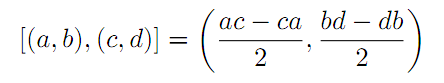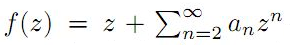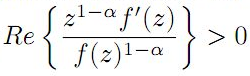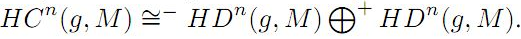The Australian Journal of Mathematical Analysis and Applications

 Home News Editors Volumes RGMIA Subscriptions Authors Contact

ISSN 1449-5910

Sorry death is imminent for file: ../public_html/searchroot/files/tex/v19n2/v19i2p4.tex
Total of 31 results found in site

6: Paper Source PDF document

Paper's Title:

On Ruled Surfaces According to Quasi-Frame in Euclidean 3-Space

Author(s):

M. Khalifa Saad and R. A. Abdel-Baky

Department of Mathematics, Faculty of Science,
KSA.
Department of Mathematics, Faculty of Science,
Sohag University, Sohag,
EGYPT.
E-mail: mohamed_khalifa77@science.sohag.edu.eg, mohammed.khalifa@iu.edu.sa

Department of Mathematics, Faculty of Science,
Assiut University, Assiut,
EGYPT.
E-mail: rbaky@live.com

Abstract:

This paper aims to study the skew ruled surfaces by using the quasi-frame of Smarandache curves in the Euclidean 3-space. Also, we reveal the relationship between Serret-Frenet and quasi-frames and give a parametric representation of a directional ruled surface using the quasi-frame. Besides, some comparative examples are given and plotted which support our method and main results.

5: Paper Source PDF document

Paper's Title:

L∞- Error Estimate of Schwarz Algorithm for Elliptic Quasi-Variational Inequalities Related to Impulse Control Problem

Author(s):

Lab. LANOS, Department of Mathematics,
P.O.Box 12, Annaba 23000,
Algeria.

Lab. LAIG, Department of Mathematics,
University May 8th 1945,
P.O.Box 401, Guelma 24000,
Algeria.

allmehri@yahoo.fr

Abstract:

In this work, we study Schwarz method for a class of elliptic quasi-variational inequalities. The principal result of this investigation is to prove the error estimate in ∞-norm for two domains with overlapping nonmatching grids, using the geometrical convergence, and the uniform convergence of Cortey Dumont.

4: Paper Source PDF document

Paper's Title:

Schwarz Method for Variational Inequalities Related to Ergodic Control Problems

Author(s):

Department of Mathematics, Badji Mokhtar University, Annaba 23000,
P.O.Box. 12, Annaba 23000, Algeria

signor_2000@yahoo.fr

Mcherihalima@yahoo.fr

Abstract:

In this paper, we study variational inequalities related to ergodic control problems studied by M. Boulbrachčne and H. Sissaoui , where the "discount factor" (i.e., the zero order term) is set to 0, we use an overlapping Schwarz method on nomatching grid which consists in decomposing the domain in two subdomains. For α ∈ ]0.1[ we provide the discretization on each subdomain converges in L -norm.

3: Paper Source PDF document

Paper's Title:

Finite and Infinite Order Solutions of a Class of Higher Order Linear Differential Equations

Author(s):

Department of Mathematics,
Laboratory of Pure and Applied Mathematics,
University of Mostaganem, B. P 227 Mostaganem,
ALGERIA

Abstract:

In this paper, we investigate the growth of solutions of higher order linear differential equations where most of the coefficients have the same order and type with each other.

2: Paper Source PDF document

Paper's Title:

Uniform Convergence of Schwarz Method for Noncoercive Variational Inequalities Simple Proof

Author(s):

Department of mathematics, LANOS Laboratory,
Faculty of the Sciences, University Badji Mokhtar,
P.O 23000 Annaba,
Algeria.

haiourm@yahoo.fr,

Abstract:

In this paper we study noncoercive variational inequalities, using the Schwarz method. The main idea of this method consists in decomposing the domain in two subdomains. We give a simple proof for the main result concerning L error estimates, using the Zhou geometrical convergence and the L approximation given for finite element methods by Courty-Dumont.

2: Paper Source PDF document

Paper's Title:

Timelike Surfaces with a Common Line of Curvature in Minkowski 3-Space

Author(s):

M.K. Saad, A.Z. Ansari, M. Akram and F. Alharbi

Department of Mathematics ,
Faculty of Science,
KSA

Abstract:

In this paper, we analyze the problem of constructing a timelike surface family from a given non-null curve line of curvature. Using the Frenet frame of the non-null curve in Minkowski space E13  we express the family of surfaces as a linear combination of the components of this frame, and derive the necessary and sufficient conditions for the coefficients to satisfy both the line of curvature and the isoparametric requirements. In addition, a necessary and sufficient condition for the given non-null curve to satisfy the line of curvature and the geodesic requirements is investigated. The extension to timelike surfaces of revolution is also outlined. Meanwhile, some representative non-null curves are chosen to construct the corresponding timelike surfaces which possessing these curves as lines of curvature. Results presented in this paper have applications in geometric modeling and the manufacturing of products. In addition, some computational examples are given and plotted.

1: Paper Source PDF document

Paper's Title:

Approximation of an AQCQ-Functional Equation and its Applications

Author(s):

Choonkil Park and Jung Rye Lee

Department of Mathematics,
Research Institute for Natural Sciences,
Hanyang University, Seoul 133-791,
Korea;

Department of Mathematics,
Daejin University,
Kyeonggi 487-711,
Korea

baak@hanyang.ac.kr
jrlee@daejin.ac.kr

Abstract:

This paper is a survey on the generalized Hyers-Ulam stability of an AQCQ-functional equation in several spaces. Its content is divided into the following sections:

1. Introduction and preliminaries.

2. Generalized Hyers-Ulam stability of an AQCQ-functional equation in Banach spaces: direct method.

3. Generalized Hyers-Ulam stability of an AQCQ-functional equation in Banach spaces: fixed point method.

4. Generalized Hyers-Ulam stability of an AQCQ-functional equation in random Banach spaces: direct method.

5. Generalized Hyers-Ulam stability of an AQCQ-functional equation in random Banach spaces: fixed point method.

6. Generalized Hyers-Ulam stability of an AQCQ-functional equation in non-Archi-medean Banach spaces: direct method.

7. Generalized Hyers-Ulam stability of an AQCQ-functional equation in non-Archi-medean Banach spaces: fixed point method.

1: Paper Source PDF document

Paper's Title:

On the Hyers-Ulam Stability of Homomorphisms and Lie Derivations

Author(s):

Department of Mathematics, Payame Noor University,
P.O. Box: 19395-3697, Tehran,
Iran.

Abstract:

Let A be a Lie Banach*-algebra. For each elements (a, b) and (c, d) in A2:= A * A, by definitions

(a, b) (c, d)= (ac, bd),
|(a, b)|= |a|+ |b|,
(a, b)*= (a*, b*),

A2 can be considered as a Banach*-algebra. This Banach*-algebra is called a Lie Banach*-algebra whenever it is equipped with the following definitions of Lie product:for all a, b, c, d in A. Also, if A is a Lie Banach*-algebra, then D: A2→A2 satisfying

D ([ (a, b), (c, d)])= [ D (a, b), (c, d)]+ [(a, b), D (c, d)]

for all \$a, b, c, d∈A, is a Lie derivation on A2. Furthermore, if A is a Lie Banach*-algebra, then D is called a Lie* derivation on A2 whenever D is a Lie derivation with D (a, b)*= D (a*, b*) for all a, b∈A. In this paper, we investigate the Hyers-Ulam stability of Lie Banach*-algebra homomorphisms and Lie* derivations on the Banach*-algebra A2.

1: Paper Source PDF document

Paper's Title:

Solving Two Point Boundary Value Problems by Modified Sumudu Transform Homotopy Perturbation Method

Author(s):

Asem AL Nemrat and Zarita Zainuddin

School of Mathematical Sciences,
Universiti Sains Malaysia,
11800 Penang,
Malaysia.
E-mail: alnemrata@yahoo.com
zarita@usm.my

Abstract:

This paper considers a combined form of the Sumudu transform with the modified homotopy perturbation method (MHPM) to find approximate and analytical solutions for nonlinear two point boundary value problems. This method is called the modified Sumudu transform homotopy perturbation method (MSTHPM). The suggested technique avoids the round-off errors and finds the solution without any restrictive assumptions or discretization. We will introduce an appropriate initial approximation and furthermore, the residual error will be canceled in some points of the interval (RECP). Only a first order approximation of MSTHPM will be required, as compared to STHPM, which needs more iterations for the same cases of study. After comparing figures between approximate, MSTHPM, STHPM and numerical solutions, it is found through the solutions we have obtained that they are highly accurate, indicating that the MSTHPM is very effective, simple and can be used to solve other types of nonlinear boundary value problems (BVPs).

1: Paper Source PDF document

Paper's Title:

Some fixed point results in partial S-metric spaces

Author(s):

M. M. Rezaee, S. Sedghi, A. Mukheimer, K. Abodayeh, and Z. D. Mitrovic

Department of Mathematics, Qaemshahr Branch,
Iran.

Department of Mathematics, Qaemshahr Branch,
Iran.
E-mail: sedghi.gh@qaemiau.ac.ir

Department of Mathematics and General Sciences,
KSA.
E-mail: mukheimer@psu.edu.sa

Department of Mathematics and General Sciences,
KSA.
E-mail: kamal@psu.edu.sa

Nonlinear Analysis Research Group,
Faculty of Mathematics and Statistics,
Ton Duc Thang University, Ho Chi Minh City,
Vietnam.
E-mail: zoran.mitrovic@tdtu.edu.vn

Abstract:

We introduce in this article a new class of generalized metric spaces, called partial S-metric spaces. In addition, we also give some interesting results on fixed points in the partial S-metric spaces and some applications.

1: Paper Source PDF document

Paper's Title:

The Higher Coefficients for Bazilevic Functions B1(α)

Author(s):

Department of Mathematics,
Faculty of Mathematics and Natural Sciences,,
Brawijaya University, Malang Jawa Timur 65145
Indonesia.
E-mail: marjono@ub.ac.id

Abstract:

Let f be analytic in D{z: |z|< 1} with, and normalized by the conditions f(0)=f'(0)-1=0. We give sharp estimates for the seventh and eighth coefficients for the class of Bazilevic functions with logarithmic growth, B1α, defined byfor α≥0.

1: Paper Source PDF document

Paper's Title:

Some Properties of (co)homology of Lie Algebra

Author(s):

Alaa Hassan Noreldeen Mohamed, Hegagi Mohamed Ali, Samar Aboquota

Department of Mathematics,
Faculty of Science,
Aswan University, Aswan,
Egypt.
E-mail: ala2222000@yahoo.com
hegagi_math@aswu.edu.eg
scientist_samar@yahoo.com

Abstract:

Lie algebra is one of the important types of algebras. Here, we studied lie algebra and discussed its properties. Moreover, we define the Dihedral homology of lie algebra and prove some relations among Hochschild, Cyclic and Dihedral homology of lie algebra. Finally, we prove the Mayer-vetories sequence on Dihedral homology of lie algebra and proved that.

1: Paper Source PDF document

Paper's Title:

Numerical Solution of Certain Types of Fredholm-Volterra Integro-Fractional Differential Equations via Bernstein Polynomials

Author(s):

Alias B. Khalaf1, Azhaar H. Sallo2 and Shazad S. Ahmed3

1Department of Mathematics, College of Science,
University of Duhok,
Kurdistan Region,
Iraq.
E-mail:  aliasbkhalaf@uod.ac

2Department of Mathematics, College of Science,
University of Duhok,
Kurdistan Region,
Iraq.
E-mail:  azhaarsallo@uod.ac

3Department of Mathematics, College of Science,
University of Sulaimani,
Kurdistan Region,
Iraq.

Abstract:

In this article we obtain a numerical solution for a certain fractional order integro-differential equations of Fredholm-Volterra type, where the fractional derivative is defined in Caputo sense. The properties of Bernstein polynomials are applied in order to convert the fractional order integro-differential equations to the solution of algebraic equations. Some numerical examples are investigated to illustrate the method. Moreover, the results obtained by this method are compared with the exact solution and with the results of some existing methods as well.

1: Paper Source PDF document

Paper's Title:

Fekete Szegö problem on the Class of Bazilevič functions B1(α) related to the Lemniscate Bernoulli

Author(s):

N. M. Asih, Marjono, Sa'adatul Fitri, Ratno Bagus Edy Wibowo

Department of Mathematics,
University of Brawijaya,
Malang 65145,
Indonesia.
Department of Mathematics,
University of Udayana,
Bali,
Indonesia.

Department of Mathematics,
University of Brawijaya,
Malang 65145,
Indonesia.
E-mail: marjono@ub.ac.id

Department of Mathematics,
University of Brawijaya,
Malang 65145,
Indonesia.

Department of Mathematics,
University of Brawijaya,
Malang 65145,
Indonesia.
E-mail: rbagus@ub.ac.id

Abstract:

We provide a sharp boundaries inequalities for Fekete Szegö problem |a3-μ a22|, the coefficients of logarithmic function log~ f(z)/z, and the coefficients of the inverse function f(f'(w)) on the Bazilevič functions B1(α) related to the Lemniscate Bernoulli on the unit disk D={z: |z| < 1}. We obtained the result by using some properties of function with positive real part relates to coefficients problems.

1: Paper Source PDF document

Paper's Title:

Walrasian Equilibrium for Set-valued Mapping

Author(s):

M. Muslikh, R.B.E Wibowo, S. Fitri

Department of Mathematics,
University of Brawijaya,
Malang,
Indonesia.
E-mail: mslk@ub.ac.id
rbagus@ub.ac.id